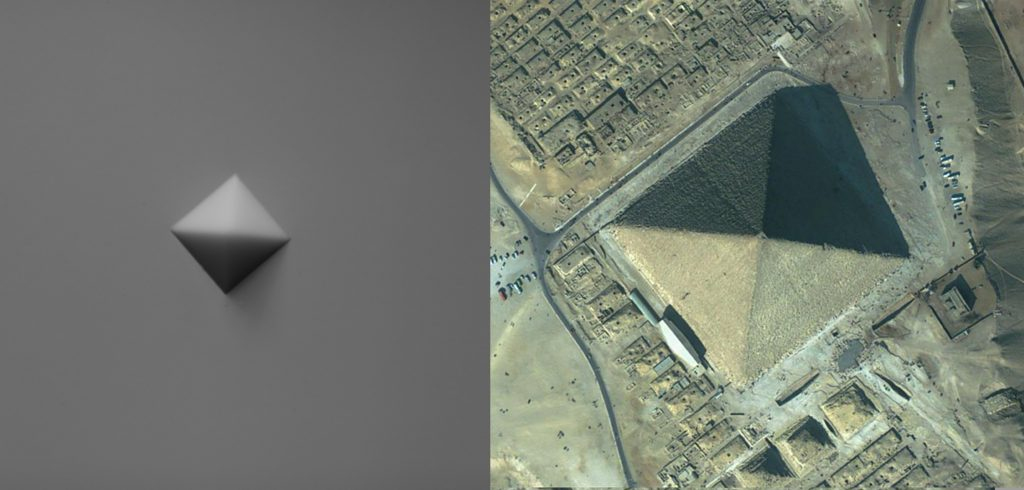# Institutional Scientific Students’ Associations Conference 2018

At this year’s Institutional Scientific Students’ Associations Conference at the Budapest University of Technology and Economics, 3 presentations were related to Morphodynamics: Krisztina Regős (1st Prize + Pro Progressio Special Prize), Dániel Csallóközi (2nd Prize + Csonka Pál Special Prize), Péter Tamás Varga (3rd Prize).

1st Prize + Pro Progressio Special Prize

Morphological analysis of fractured rock surfaces
Krisztina Regős
supervisors: Gábor Domokos, Zsolt Lángi, Norbert Krisztián Kovács

In 3-dimensional space, solids with flat polygonal faces are called polyhedra. We call a polyhedron P convex if the line segment between any two points of P is contained in P. Cubes and tetrahedra are examples of convex polyhedra.

We may classify polyhedra based on the numbers f of faces, e of edges and v of vertices and Euler proved that for any convex polyhedron we have f+v=e+2. Using Euler’s Theorem we can define the primary combinatorial classes (f,v)^C containing convex polyhedra with f faces and v vertices.

Another, probably less known, but rather intuitive classification of polyhedra is based on the number of their mechanical equilibrium points. The latter may belong to three stability types: on faces they are called stable (S), on vertices they are called unstable (U) and on edges they are called saddle-type (H) equilibria. The Poincaré-Hopf formula shows for a convex polyhedron that S+U=H+2, so convex polyhedra can be classified into (S,U)^E primary equilibrium classes.

Beyond the inequalities f≥S, v≥U there is very little known about the general relation between the two classification systems and there are many open questions, even in classes which appear to be simple.

In my paper I provide the (S,U)^E classification of polyhedra in the primary combinatorial class (5,5)^C (called pentahedron or pyramid) by creating and using a MATLAB code. I was seeking the answer to the following questions:

(a) CONJECTURE (J.H. Conway, 1969): Pentahedra do not exists in primary equilibrium classes (S,1)^E, (1,U)^E (S,U=1,2,3,4,5).
(b) CONJECTURE: Pentahedra exist in all primary equilibrium classes (S,U)^E (S,U=2,3,4,5).

With the help of my program I got positive answer to both questions, namely I confirmed numerically the 1969 conjecture of J.H. Conway and I provided a constructive proof for Conjecture (b). To further support the last statement, I created one pentahedron in each of the 16 identified classes with 3D printer.2nd Prize + Csonka Pál Special Prize

Numerical Analysis on Distance-Driven Shape Evolution of Ellipsoids
Dániel Csallóközi
supervisor: Gábor Domokos

The first known description about the shape evolution (abrasion) of objects found in nature (pebbles) originates from Aristotle. The mathematical model based on his idea is a so-called radial distance-driven model, of which we know that it seldom appears in nature.

However, the study of shape evolution (morphodynamics) is currently an actively researched area. Other possible models of these processes, similar to Aristotle’s, are the parallel distance-driven models, including orthogonal affinity.

In these models, the abrasion of a pebble can be visualised as the surface points of the pebble move towards the inside of the pebble according to some rule. In orthogonal affinity, is given a plane, constant in time and intersecting the original figure. The surface points of the figure move towards the plane on straight lines perpendicular to the plane. The motion of any surface point is the function of only the distance between the point and the plane.

In geology, the most commonly used shape evolution descriptors are the axis ratios of the figure, defined with a specific method, and the isoperimetric ratio, which describes the relation between the volume and the surface of the figure.

In previous studies , the alteration of axis ratios of a triaxial ellipsoid under orthogonal affinity in axial direction is proved. However, for orthogonal affinity in a general direction, only conjectures are drawn. The alteration of the isoperimetric ratio in general cases is concluded and proved.

With my study, I aim illustrating the proved theorems, analysing the conjectures via numeric methods, and I visualise the process in three-dimensions.

Creating this study, I used parts of curriculum of the optional subject “Mathematical Modelling of Mechanical Problems”, and my previous (2017) Scientific Student Conference (TDK) study.

 Domokos, G. & Lángi, Z., The Evolution of Geological Shape Descriptors Under Distance Driven Flows, Math Geosci (2018) 50: 337.
https://doi.org/10.1007/s11004-017-9723-9

3rd Prize

How complex is a symplex?
Tamás Péter Varga
supervisors: Gábor Domokos, Zsolt Lángi, Norbert Krisztián Kovács

Among the three-dimensional bodies, the tetrahedron has a prominent position, which can be regarded as the simplest body type in many ways. The concept of tetrahedras is widely known, we could imagine a regular polyhedron with 4 regular triangles in an instance.

However, by renouncing regularity, the world of tetrahedras becomes surprisingly complicated.

In addition to geometric forms, we can think of three-dimensional symplexes as solid physical bodies, and in this approach that complexity becomes tangible: it can be proved (partly based on the work of JH Conway) that based on the primary equilibrium classification, determined by the number of stable and unstable mechanical equilibria (S, U), there can be 9 type of tetrahedras.

The purpose of my dissertation is to demonstrate by constructive means the existence of these 9 types. The proof is to map the compact 5-dimensional configuration space of the tetrahedrals by numeric method in the MATLAB environment, and the classification of the tetrahedrals identified in the grid points of the configuration space into the primary equilibrium classes and the determination of their mechanical complexity.